# MATLAB Assignment 1

Math 473 Fall 2020
You learned in class the Euler method for approximating a solution to the differential equationGiven an initial condition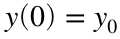, the method gives a sequence of approximations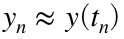using the recursion scheme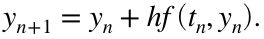where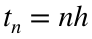and h is the time step chosen by the user.

## Warmup

A simpler recursion relation would look like thisWe know the exact solution to this recurrence isso we plot this as well;
N = 10;
n=0:N;
y = zeros(1,N+1);
y(1) = 0;
for j=1:N
y(j+1) = y(j) + j;
end
yExact=n.*(n+1)/2;
plot(n,y,'o',n,yExact,'x')
xlabel('n');ylabel('y')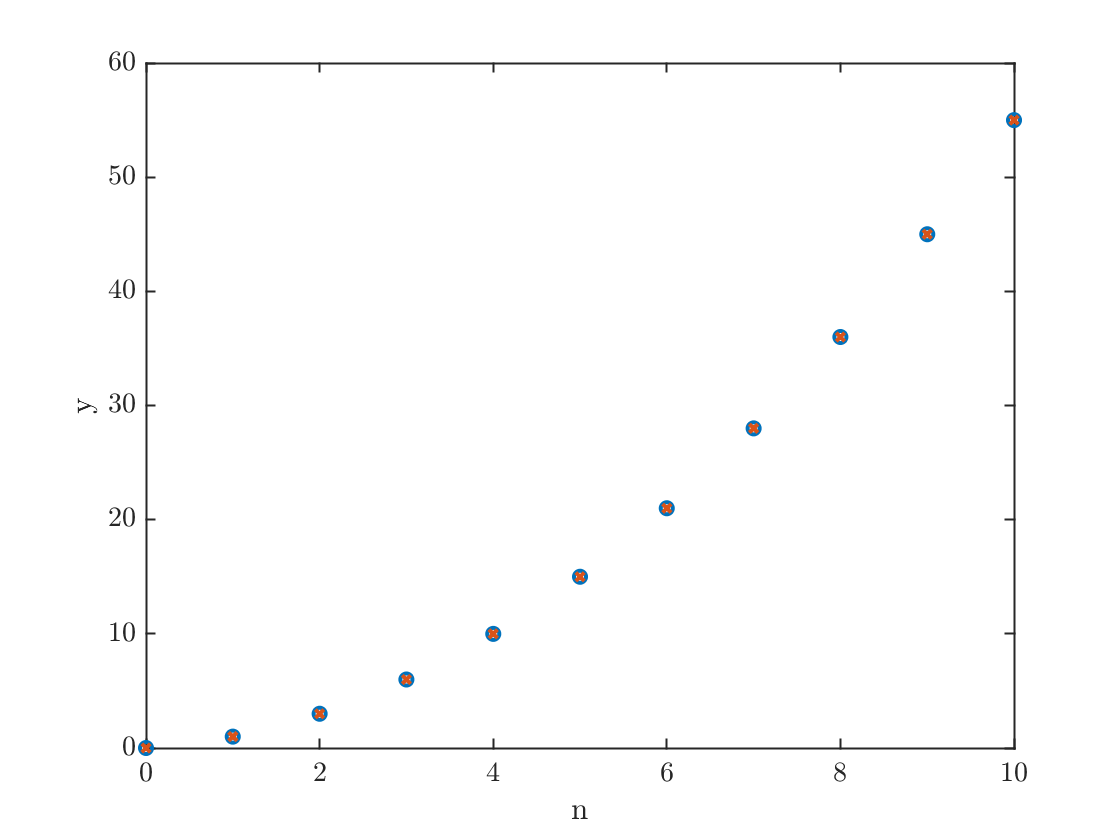## The Euler method

Let's solve the problemThis has exact solution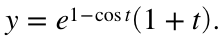tFinal = 2*pi;
N = 100;
h=tFinal/N;
t=linspace(0,tFinal,N+1); % type 'help linspace' to see how this works
y=zeros(1,N+1);
yExact=exp(1-cos(t)).*(1+t);
I want to point out the syntax used to define yExact in the above line. In particular the .* notation which is used for elementwise multiplication, since exp(1-cos(t)) and (1+t) are each vectors. You will need this to form the exact solution below, you will also need to use the .^ notation to compute an elementwise power.
y(1) = 1; % setting the initial condition
for n=1:N
y(n+1) = y(n) + h * (sin(t(n))+1/(1+t(n)))*y(n);
end
plot(t,y,t,yExact,'--'); xlabel('t'); ylabel('y'); title('Look, ma! I solved an ODE in MATLAB!');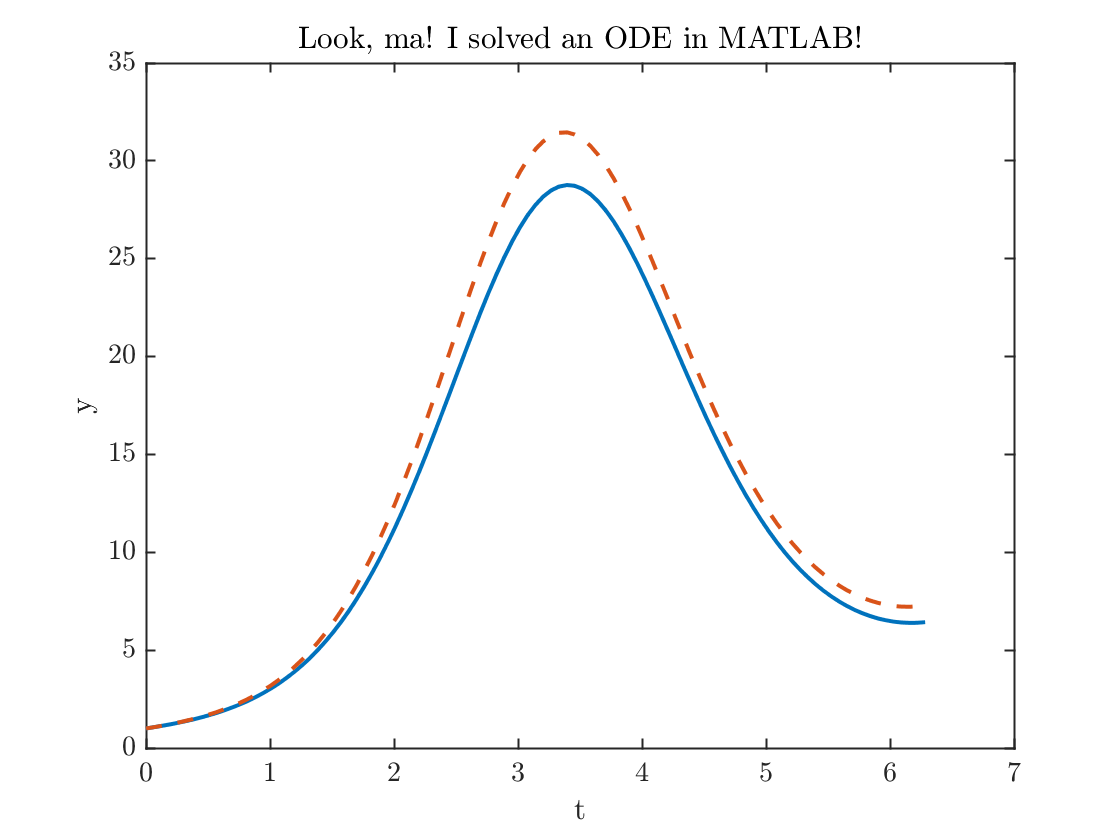error100= abs(y(N+1)-yExact(N+1));
fprintf('The error at the final time = %0.2f.\n',error100)
The error at the final time = 0.85.
That error is a little bit larger than I'd like, let's double the number of points and try again
N = 200;
h=tFinal/N;
t=linspace(0,tFinal,N+1); % type 'help linspace' to see how this works
y=zeros(1,N+1);
yExact=exp(1-cos(t)).*(1+t);
y(1) = 1; % setting the initial condition
for n=1:N
y(n+1) = y(n) + h * (sin(t(n))+1/(1+t(n)))*y(n);
end
plot(t,y,t,yExact,'--')
xlabel('t'); ylabel('y'); title('Look, ma! I solved it even better!');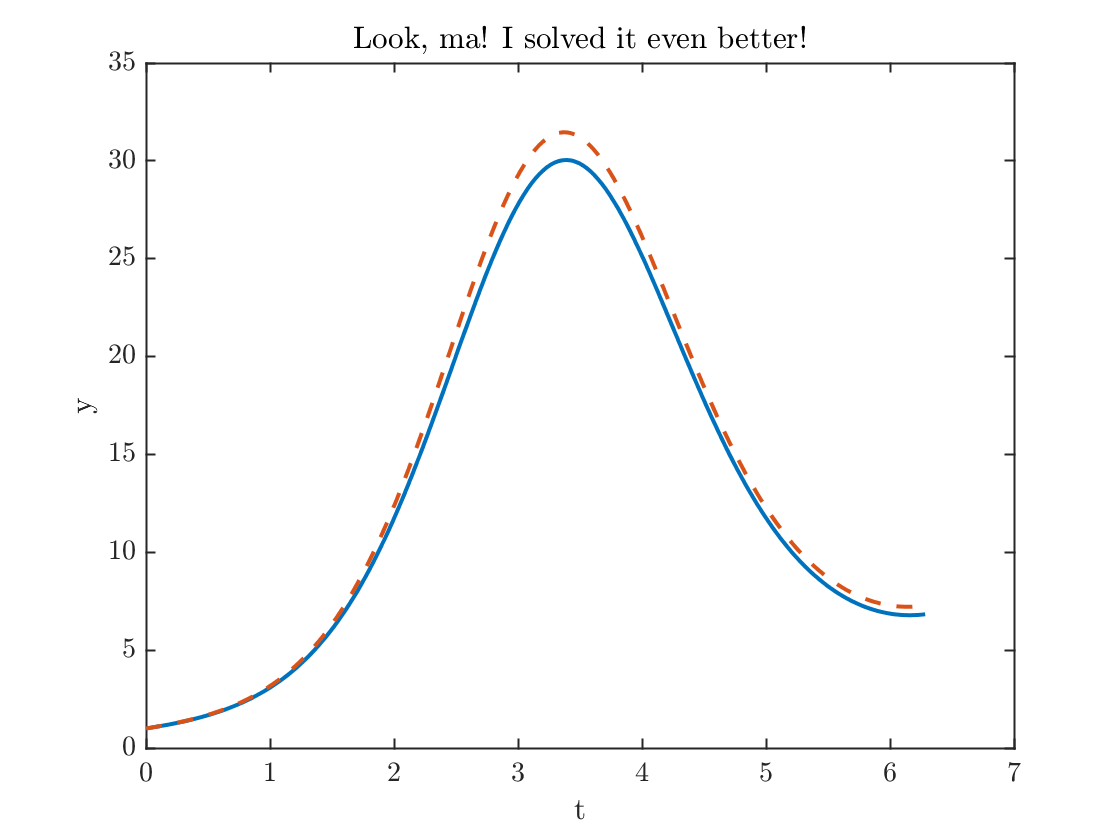error200= abs(y(N+1)-yExact(N+1));
fprintf('The error went down by a factor of %f.\n',error100/error200);
The error went down by a factor of 1.906908.

## Using a function

Note how the differential equaiton is hardcoded inside the for-loop. This is a terrible idea. (Can you see why?) It is better to define the differential equation inside a function. At the bottom of this file, is a function f. We can solve the differential equation that calls this function instead
z=zeros(1,N+1);
z(1)=1;
for n=1:N
z(n+1) = z(n) + h* f(t(n),y(n));
end
plot(t,z)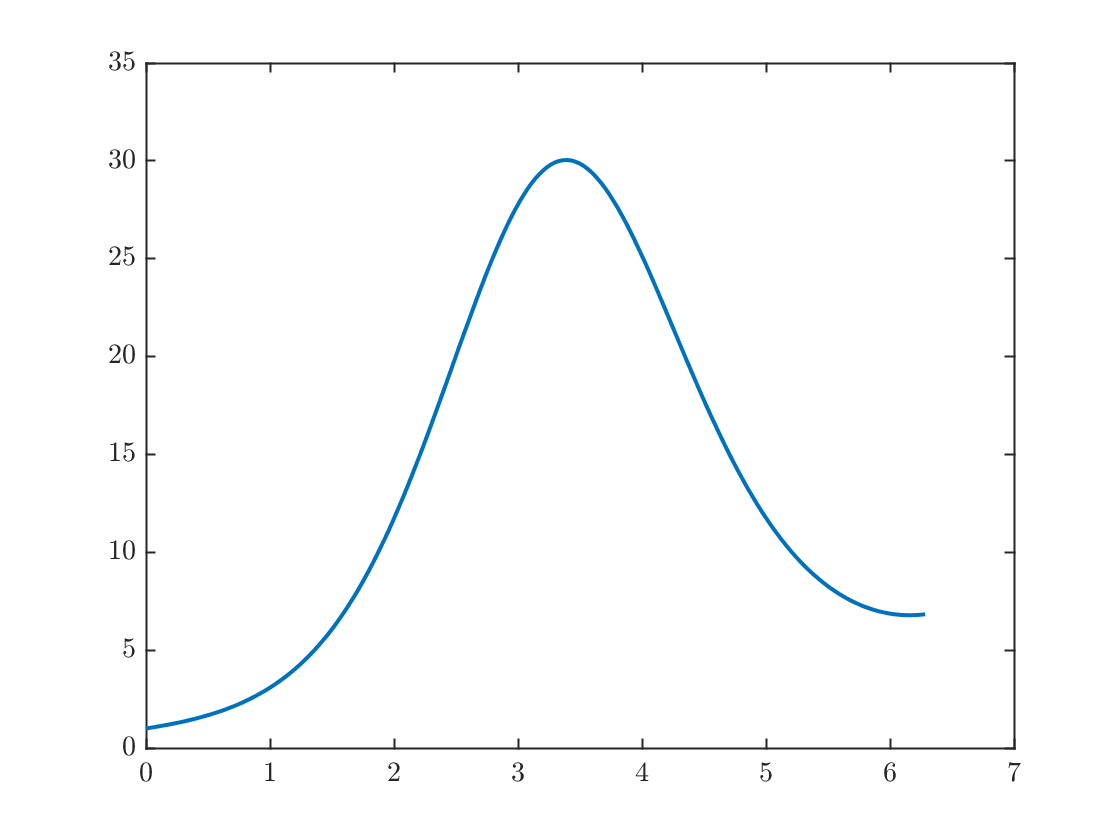Question 1: Apply the Euler method to solvefrom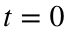to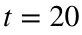. (Note that the equation is not defined at, so we have to start at a different initial time. Use a function as we used to find z(t) in the most recent section.
Cut and paste the above snippet of code into the MATLAB editor. Modify as necessary and save it as a .m file in order to do the following:
Note that this problem has exact solutionwhich is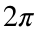-periodic, so that the value at the final time is is just. It is also easy to see how badly the method fails when you take N too large.
Then do the following:
• Figure out what is the minimum value of N that you need to get an error smaller than 0.01 at the final time. Do this by experimenting with N until you get a small enough error.
• Plot the computed and exact solution using this value of N.
• Show the method is approximately second order by doubling N and computing the ratio of the errors.
• Hint: MATLAB does not have the value of Euler's number e built in, but it does have the exponential function, i.e.t to evaluate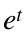, use the command exp(t), so that to get e, type exp(1).
You should hand in a printout of your code, any figures you print, and a report of a few sentences answering all the questions.
Question 2 The Euler method is an example of a first order method. This means that the error is proportional to h, so that doubling the number of points roughly cuts the error roughly by a factor of two.
The improved Euler method is what's known as a two-step method. It requires two evaluations of the function per time step. At each step, we define two extra quantities before computing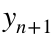.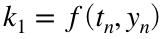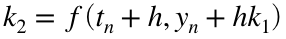The payoff for using this more complicated method is that it is second order! This means that each time you double the number of time steps the error goes down by a factor of four. This is a big deal.
Program this method to solve the same problem from Question 1 and
• Determine how large N needs to be get an error less than 0.01.
• Show the method is second order by doubling N and seeing that the error has gone down by a factor of four.
• Here we see the payoff of defining f(t,y) in a separate function.
Question 3 In practice, scientists are most likely to use the fourth order Runge-Kutta method for time stepping. This requires four computations per step.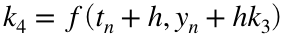The payoff for using this more complicated method is that it is fourth order! This means that each time you double the number of time steps the error goes down by a factor of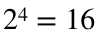. This is a really big deal.
Program this method to solve the same problem from Question 1 and
• Determine how large N needs to be get an error less than 0.01.
• Show the method is fourth order by doubling N and seeing that the error has gone down by a factor of sixteen.
• Again, use a function to define the right hand side.
For certain applications, such as computing spacecraft trajectories, scientists are likely to use methods that are of much higher order, even 12th order!
function yprime=f(t,y)
yprime = (sin(t)+1/(1+t))*y;
end